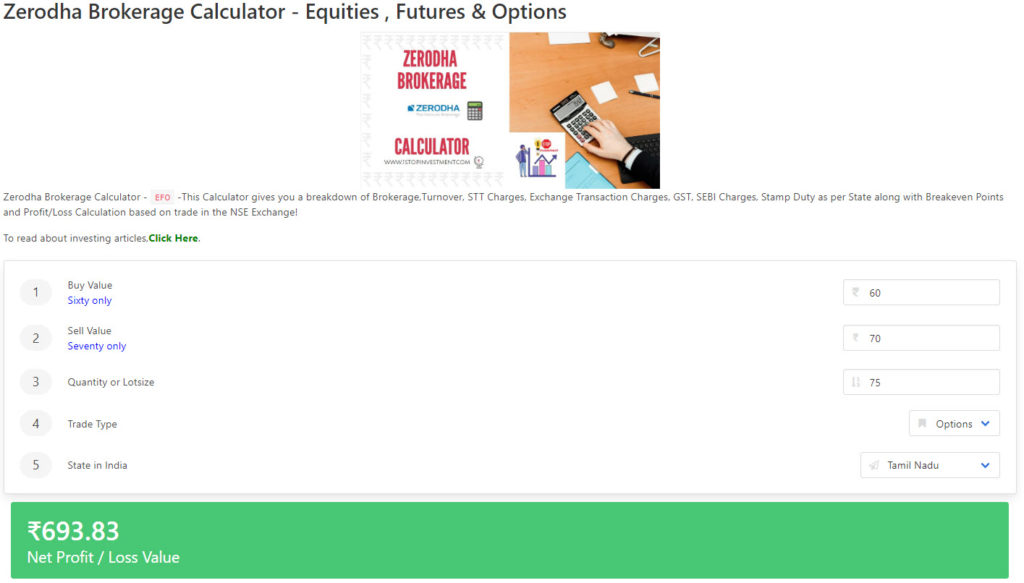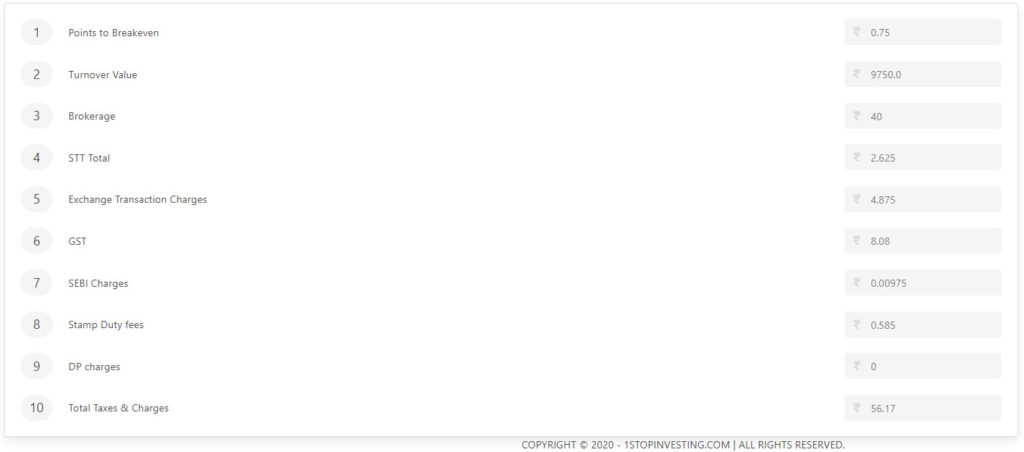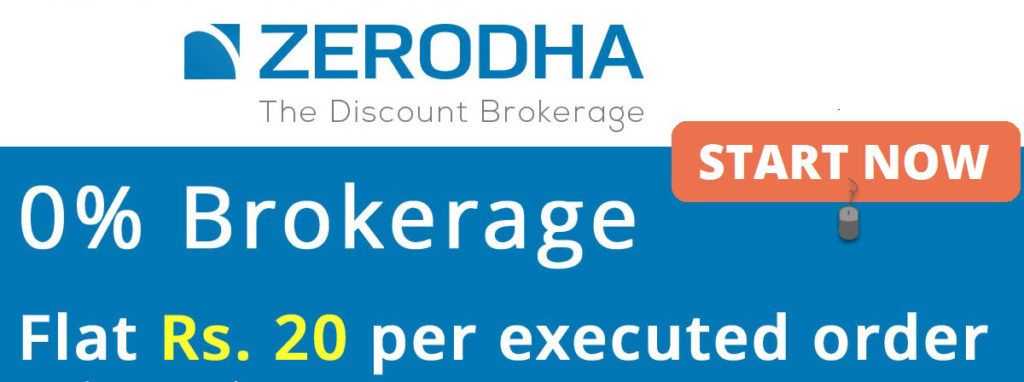## How do Zerodha Brokerage charges works in Equity, Future & Options?

Are you looking for any below ?

## Who is Zerodha ?

As of 2019, Zerodha is the largest retail stock broker in India by active client base.

Zerodha is an Indian financial service company (member of NSE, BSE, MCX, MCX-SX), that offers retail and institutional broking, currencies and commodities trading, mutual funds, and bonds. Founded in 2010, Zerodha is known for its discount pricing model and technology. It is headquartered in Bangalore and has physical presence in all major Indian cities.

## What are the Charges for Equity Intraday?

BROKERAGE: 0.01% or Rs. 20/executed order whichever is lower.

• Per Executed order means – 1 for buy and 1 for Sell = 2 for one trade.
• For mutliple trades, brokerage applies for each executed order.

STT/CTT : 0.025% on the sell side

Exchange Transaction Charges : NSE: 0.00325% & BSE: 0.003%

GST :  18% on (brokerage + transaction charges)

SEBI CHARGES :  ₹10 / crore

STAMP CHARGES : It Varies on State to State, For Tamilnadu, it is 0.006% of the turnover.

Call & trade : Additional charges of ₹20 per order if Orders placed through support desk and Intraday positions squared off by their RMS team.

### Let me explain with an example:

For the Calculation purpose, Assuming that , you bought  an Equity Stock at 546 and Sold at 550 in the same day with the traded Quantity of 100 nos.

Before proceeding into calculation,

What is Turnover value :

If you buy 100 stocks at Rs 546 and sell at Rs 550, total turnover value is (546*100+ 550*100=) Rs 1,09,600.

• BROKERAGE: 0.01% or Rs. 20/executed order whichever is lower.
• Brokerage = 0.01%*109600 = Min(10.96 ,20*2 ) = Rs. 10.96.
• STT  = 0.025%*(550*100) = 13.75-> rounded to 14.
• Exchange Transaction Charges : 0.0325% * 109600 = 3.56
• GST =  18% *(10.96 + 3.56) = 2.61
• SEBI charges = 109600/1crore * 10 = 0.11
• Stamp Charges = 0.006% * 109600 =6.58
• Total Charges = ₹37.82
• Net P&L = ₹362.18

## What are the Charges for Equity Delivery?

BROKERAGE: 0%.

STT/CTT : 0.1% on buy & sell

Exchange Transaction Charges : NSE: 0.00325% & BSE: 0.003%

GST :  18% on (brokerage + transaction charges)

SEBI CHARGES :  ₹10 / crore

STAMP CHARGES : It Varies on State to State, For Tamilnadu, it is 0.006% of the turnover.

### Let me explain with an example:

For the Calculation purpose, Assuming that , you bought  an Equity Stock at 546 taken delivery and Sold at 550 in the other day with the traded Quantity of 100 nos.

Before proceeding into calculation,

What is Turnover value :

If you buy 100 stocks at Rs 546 and sell at Rs 550, total turnover value is (546*100+ 550*100=) Rs 1,09,600.

• BROKERAGE: 0
• Brokerage = 0
• STT  = 0.1% *(546*100) +0.1%*(550*100) = 110
• Exchange Transaction Charges : 0.0325% * 109600 = 3.56
• GST =  18% *(0 + 3.56) = 0.64
• SEBI charges = 109600/1crore * 10 = 0.11
• Stamp Charges = 0.006% * 109600 =6.58
• Total Charges = ₹136.82
• Net P&L = ₹263.18

## What are the Charges for Equity Futures?

BROKERAGE: 0.01% or Rs. 20/executed order whichever is lower

STT/CTT : 0.01% on the sell side

TRANSACTION CHARGES : NSE: 0.0019%

GST :  18% on (brokerage + transaction charges)

SEBI CHARGES :  ₹10 / crore

STAMP CHARGES : It Varies on State to State, For Tamilnadu, it is 0.006% of the turnover.

Call & trade : Additional charges of ₹20 per order if Orders placed through support desk and Intraday positions squared off by their RMS team.

### Let me explain with an Nifty Future trade:

For the Calculation purpose, Assuming that , you bought  an Nifty Future at 12000 and Sold at 12100  with the traded Quantity of 1lot (=75nos).

Turnover value :(12000*75+ 12100*75=) Rs 18,07,500

• BROKERAGE: 0.01% or Rs. 20/executed order whichever is lower.
• Brokerage = 0.01%*18,07,500 = Min(180.75 ,20*2 ) = Rs. 40
• STT  = 0.01%*(12100*75) = 90.75-> rounded to 91.
• Exchange Transaction Charges : 0.0325% * 18,07,500= 34.34
• GST =  18% *(40 + 34.34) = 13.38
• SEBI charges = 18,07,500/1crore * 10 = 1.81
• Stamp Charges = 0.006% * 18,07,500 =108.45
• Total Charges = ₹288.98
• Net P&L =₹ 7211

## What are the Charges for Equity Options?

BROKERAGE: Flat Rs. 20 per executed order

STT/CTT : 0.05% on the sell side (on premium)

TRANSACTION CHARGES : NSE: Exchange txn charge: 0.05% (on premium)

GST :  18% on (brokerage + transaction charges)

SEBI CHARGES :  ₹10 / crore

STAMP CHARGES : It Varies on State to State, For Tamilnadu, it is 0.006% of the turnover.

Call & trade : Additional charges of ₹20 per order if Orders placed through support desk and Intraday positions squared off by their RMS team.

### Let me explain with an Nifty Options trade:

For the Calculation purpose, Assuming that , you bought  an Nifty Call Options 12000CE at 60 and Sold at 70 with the traded Quantity of 1lot (=75nos).

Turnover value :(60*75+ 70*75=) Rs 9,750

• BROKERAGE: Flat Rs. 20 per executed order
• Brokerage = (20*2 ) = Rs. 40
• STT  = 0.05%*(70*75) =2.62-> rounded to 3.
• Exchange Transaction Charges : 0.0325% * 9,750=4.88
• GST =  18% *(40 + 34.34) =8.08
• SEBI charges = 18,07,500/1crore * 10 = 0.01
• Stamp Charges = 0.006% * 9,750 =0.58
• Total Charges = ₹56.17
• Net P&L =₹ 693.83

Thanks for reading and Share it with your friends if you find it useful.

The above calculation is embedded into ourVisit Zerodha Website and fill your details to setup your trading account easily. You can click here to visit Account Opening PageShare with your friends if you like